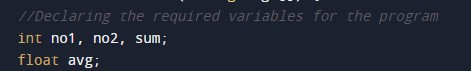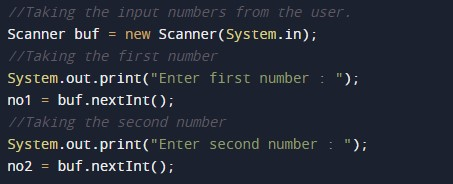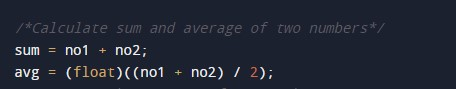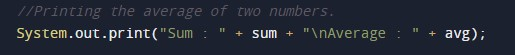# Java Program to Calculate Average of Two Numbers

In this tutorial you will learn about the Java Program to Calculate Average of Two Numbers and its application with practical example.

## Java Program to Calculate Average of Two Numbers

In this tutorial, we will learn to create a Java program that will Calculate the Average of Two Numbers in Java programming.

## Prerequisites

Before starting with this tutorial, we assume that you are the best aware of the following Java programming topics:

• Operators in Java Programming.
• Basic Input and Output function in Java Programming.
• Basic Java programming.
• Arithmetic operator in Java programming.

## What is the average of the numbers?

The average of numbers in mathematics means that all the numbers are added and then the sum of all the numbers is divided by the total number of objects.

It means if there are 4 numbers as follows {2,4,6,8} then the average will be:-

average = 2+4+6+8/4

average = 5.

## Program to Calculate the average of a Number

In this program, we will take two numbers in input from the user. Then we will add that number and store it in a variable. After that, we will divide the sum of those numbers by 2 to find the average. At last, we will print the average of two numbers.

With the help of this program, we can find the average of two numbers.

## Output:-In the above program, we have first initialized the required variable.• no1 = it will hold the integer value.
• no2  = it will hold the integer value.
• sum = it will hold the integer value.
• avg = it will hold the float value.

Taking input numbers from the user to for the average of two numbers.Calculating the average of those numbers.Printing output average for that given number.In this tutorial we have learn about the Java Program to Calculate Average of Two Numbers and its application with practical example. I hope you will like this tutorial.